• If you are citizen of an European Union member nation, you may not use this service unless you are at least 16 years old.

• You already know Dokkio is an AI-powered assistant to organize & manage your digital files & messages. Very soon, Dokkio will support Outlook as well as One Drive. Check it out today!

View

# Chemical Equilibrium 2014

last edited by 8 years, 12 months ago

Introduction:

When a chemical reaction appears to end, often this does not mean that all reactants have been consumed. Rather, the system approaches a state where the reactants and products are present in fixed relative amounts, called equilibrium. Chemical equilibrium is determined by a number of factors, with Gibbs free energy and chemical potential being the most notable representatives for the way in which a certain reaction will equilibrate under given conditions. Multiple system conditions will affect these terms, and understanding the way in which this happens and the meaning behind the equations will allow one to more fully understand how to predict reactions and what it means for a system to be at equilibrium.

What is equilibrium?

Equilibrium is the state of a system where the reaction has seemingly ended. However, the reaction has not “stopped” - rather, the forward and reverse reactions have balanced out so that, while reaction is ongoing, the relative amounts of reactants and products are equal. Thus, a good formal definition of equilibrium would be that it is the state where there is no net conversion of reactants to products (or vice versa) unless the experimental conditions of the reaction are changed1(p616).

Gibbs Free Energy

Gibbs free energy is a state function that represents the feasibility of a reaction in a system based on the enthalpy, temperature, and entropy. Gibbs free energy is calculated at constant temperature and pressure. The change in Gibbs free energy is the most relevant information, as the sign of this number gives information about the direction of a reaction.

The actual calculation for Gibbs free energy is given as

G = H - TS

where G is the Gibbs free energy (in joules), H is the enthalpy (in joules), and TS is temperature (in Kelvin) times entropy (in joules per Kelvin).

Using this, we can find the change in Gibbs free energy, ΔG:

ΔG = ΔH - TΔS

What does the change in Gibbs free energy tell us? Let’s say we have the reaction A → B. If we know that ΔG < 0 for this reaction, we know that the reaction is spontaneous proceed from A to B, as it is favorable for ΔG to be negative - Gibbs free energy is always minimized. This means that the product B is preferred. If, however, ΔG > 0, then the reaction will spontaneously proceed in the reverse direction - meaning that A → B is not spontaneous but B → A is. If ΔG = 0, then the reaction is in equilibrium and there is no net change in the amount of A or B.

The molar Gibbs energy at a given temperature and pressure is called chemical potential, μ: it represents the potential energy that can be absorbed or released during a chemical reaction.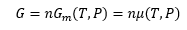The change in Gibbs free energy can be easily calculated for an ideal gas based on pressure change. If the pressure of a gas isothermally changes from P1 to P2 we can rewrite ΔS asbased on the relationship between volume and pressure per the Ideal Gas Law. Since ΔG is measured at constant temperature and we are examining an isothermal pressure change of an ideal gas, we can also say that ΔH is equal to zero. This means that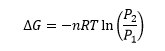In order to calculate the chemical potential, all one has to do is calculate this change in Gibbs free energy per mole. To standardize this, the change in Gibbs free energy is calculated between the final pressure P and some reference pressure (usually standard atmospheric pressure of 1 atm, so that the change in chemical potential can be more easily expressed). This leaves us with our final equation as follows: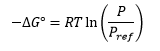Equilibrium condition

Gibbs free energy and chemical potential are just two different perspectives for us to study the chemical equilibria. Defined as the molar Gibbs free energy (constant pressure), chemical potential is essentially presenting the same concept as Gibbs energy; however, chemical potential can be directly calculated from a simple balanced reaction equation. In 1877 van't Hoff showed that it is a function of the concentration of the various species involved, and that the concentrations appear as powers corresponding to the stoichiometric number (the coefficients of each substance) in the balanced chemical equation. For example, in the reaction, chemical potential can be written as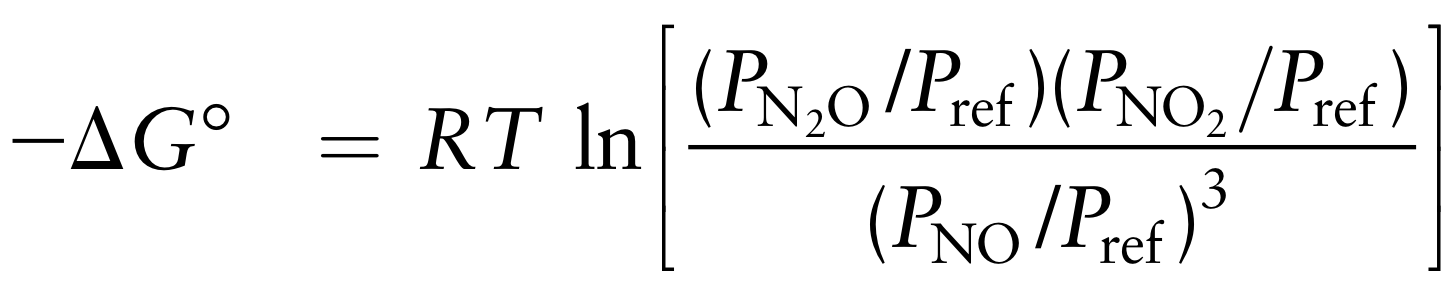Since ∆G = 0 under equilibrium, we have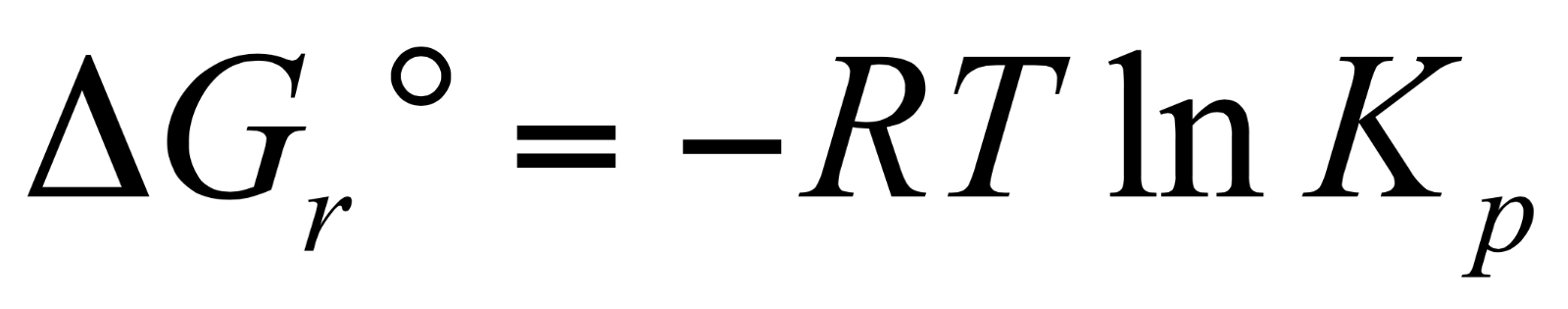from the initial equation. To actually calculate the chemical equilibrium constant, for example in a balanced chemical reaction equation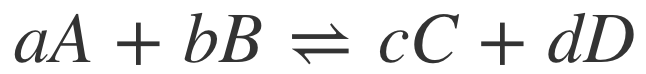, we can calculate the equilibrium constant K since the stoichiometric numbers give us the partial pressure.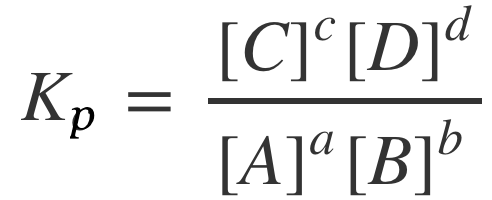After that we can plug Kp back to the -∆Gº equation and get the standard Gibbs free energy for the reaction we have.

Equilibrium Constant vs. Reaction Quotient

As seen above, you can see how the equilibrium constant, K was obtained. From that you should understand that, K represents the equilibrium constant and is often used to find the concentrations of each component of a reversible reaction at equilibrium. The equilibrium constant is known to be related to the reaction quotient but their differences are very important to understand. The reaction quotient ‘Q’, for gas-phase reactions is defined as the amount of reactants and products present in a reaction during a specific point of time and aids in figuring out which direction the reaction is likely move in.

The difference between the two is that K (equilibrium constant) is a constant dependent on temperature and can be determined by the partial pressures of the products and reactants of a reaction at equilibrium. Whereas the Q (reaction quotient) changes all the time due to its dependence on the instantaneous partial pressures of the reaction. In contrast of their differences K and Q are related in a way that when a reaction approaches equilibrium Q approaches K. The reason for this is because the initial partial pressures, PA, PB, PC, PD give an initial reaction quotient of Q0, which is relative to K and determines the reactions spontaneous direction toward equilibrium. This means that as time goes on Q must increase only if Q0 is less than K which results in the reaction going from left to right. Inversely, if Q0 is greater than K then Q must decrease until equilibrium is met which results in the reaction going from right to left. Below is an illustration to help you get a better visual and understanding of this concept.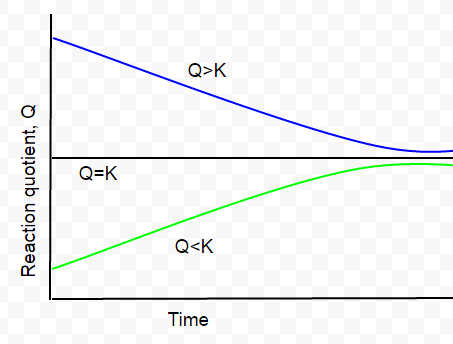Figure: “If the initial reaction quotient Q0 is less than K it increases with time; if it is greater than K, it decreases.”1

Difficult Concept: Le Chatelier Principle

Many Chem 260 students have a difficult time grasping the Le Chatelier Principle concept. The Le Chatelier Principle “provides a way to predict quantitatively the direction of change of a system under an external perturbation”1 Reactions in equilibrium can shift due to an impact by physical changes (i.e.pressure, temperature, and temperature). For example if the pressure of the following reaction vessel, aA(s) +bB(g) ⇌  cC(g) + dD(g) is decreased then this will cause the reaction to go towards/ favor the products. In addition to this if the pressures of the reactants and products are decreased in a reaction then this would cause a shift towards the side of the reaction with the highest moles of gas. If the pressures of the reactants and products were increased then it would cause a shift towards the side of the reaction with the lowest moles of gas.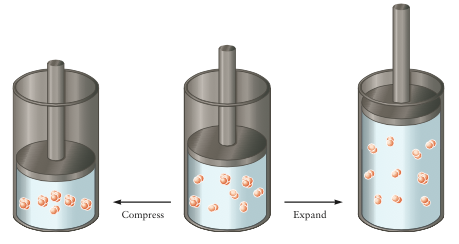Figure 1: The cylinder in the middle is contained with an equilibrium mixture of P2 and P4. When the cylinder is compressed (left cylinder) then some of the P2 molecules combine to make P4, which reduces the total number of molecules and total pressure. When the cylinder is expanded to increase the volume, then “some P4 molecules dissociate to pairs of P2 molecules to increase the toal number of molecules and the pressure exerted by those molecules.”1

In terms of an impact of concentration on a reaction in equilibrium, if more species of the product is added to the reaction vessel then the reaction will shift to favor the reactants of the reaction. Inversely, if more species of the reactant is added to the reaction vessel than the reaction will favor to the products.

A reaction that is impacted by temperature can have a heat component on the products and reactant side of the equation depending on if the reaction is endothermic or exothermic. A shift towards the reactants will only occur if the reaction is exothermic and the temperature is raised. If the temperature is lowered in an exothermic reaction then the products will be favored. Conversely, if a reaction is endothermic and the temperature is raised then it will cause a shift towards the products but if the temperature is lowered then it will cause a shift towards the reactants.

The Le Chatelier’s  Principle is often used in biochemistry with the use of catalysts or enzymes to speed up reactions or shift the equilibriums of a reaction depending on how forward or backwards they are impacted from the physical changes. This is why understanding the Le Chatelier Principle is so important.

Concept Questions

Question 1: True or false: A system at equilibrium has no net conversion of reactants to products

A. True - equilibrium means that the forward and reverse reactions are balanced

B. True - equilibrium means that the reaction has completed and is all product

C. False - equilibrium means that the reaction rate is constant so the reaction will continue

D. False - because entropy is always greater than zero equilibrium can never be reached

Question 2: For a given reaction A→B, we know that ΔG < 0. Based on this we can say:

A. The product B is preferred

B. The reactant A is preferred

C. The reaction is spontaneous

D. A and C

E. B and C

Question 3: consider the discussion on chemical potential above, which one of the following is true:

A. chemical potential can be obtained from the stoichiometric numbers from a chemical reaction equation;

B. chemical potential can show whether a reaction has reached the equilibrium;

C. chemical potential equals to molar gibbs free energy only under constant pressure and temperature

D. both B & C

E. A, B & C

Question 4: calculate Kp for the following reaction, the partial pressure are 195 Torr, 265 Torr, 180 Torr and 260 Torr, respectively.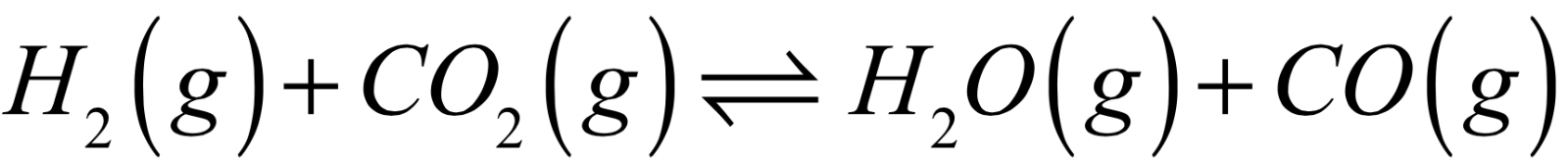Question 5: Given the reaction at equilibrium: 2CO(g) + O2(g) <-> 2CO2(g) When the reaction is subjected to stress, a change will occur in the concentration of A. reactants, only

B. products, only

C. both reactants and products

D. neither reactants and products

Question 6: Consider the following equilibrium:

2 SO2(g) + O2(g) ⇌  2SO3(g) + heat

Which change will shift the equilibrium to the right?

A. decreasing the pressure

B. increasing the temperature

Citations:

  Oxtoby, David W., H. P. Gillis, and Alan Campion. Principles of Modern Chemistry. 7th ed.

Cengage Learning, 2011. Print.

 Voigt, H. (n.d.). The Equilibrium Constant. Retrieved November 29, 2014, from http://chemwiki.ucdavis.edu/Physical_Chemistry/Equilibria/Chemical_Equilibria/The_Equilibrium_Constant

http://www2.mcdaniel.edu/Chemistry/ch307.notes/Chemical%20Equilibrium.html

http://chemwiki.ucdavis.edu/Physical_Chemistry/Equilibria/Chemical_Equilibria/The_Equilibrium_Constant/Balanced_Equations_And_Equilibrium_Constants_2

1. A - chemical equilibrium is a dynamic equilibrium in which the forward and reverse reactions proceed at the same rate. Because of this, it is accurate to say that the net amount of reactants and the net amount of products are both constant, as reactants are being consumed as fast as they are being reproduced.

2. D - The sign of the change in Gibbs free energy, ΔG, determines the direction of the reaction. Gibbs free energy is always minimized, so if ΔG < 0 for a reaction, the products of that reaction are favored over the reactants, and the reaction will proceed spontaneously. That means both A and C are correct, so D is the final answer.

3. D

4. Kp = 1.1

5. C - According to the Le Chatelier’s Principle, if a change (‘stress’) in temperature or concentrations is added to a system at equilibirum then the reaction will shift in a way that uses up that change (‘stress’). So, if more CO(g) and O2 are added to the system then more CO2(g) would result. In constrast, if more CO2(g) product is added to the system then the concentration of the reactants would increase by using up the excess product changing it back to CO(g) + O2(g).

6. D - To get more of the product [SO3(g)] there would be an addition of more reactants. As the reaction reaches equilibrium, the extra reactants are being converted into the product [SO3(g) + heat] causing the reaction to shift to the right.